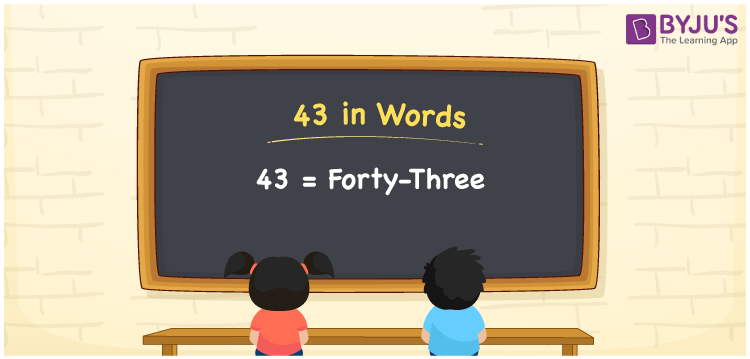# 43 in Words

We can write 43 in Words as Forty-Three. In the number system, 43 is a number that shows a value equivalent to it. For example, Sheetal has bought a notebook for Rs. 43. Using words, we can represent in the sentence as “Sheetal has bought a notebook for Rupees Forty-Three”. Hence, it is essential for students to learn how to write and read the number names. 43 is a cardinal number since it shows a specific quantity. Let us learn how to write 43 in words in this article.

 43 in words Forty-Three Forty-Three in numerical form 43

## 43 in English Words

Generally, students are taught to write numbers in words using the English language right from primary classes. In the education field, English is the most commonly used language. Therefore, students must learn English to know how to read and write number names. Hence, 43 in words is written as Forty-Three.## How to Write 43 in Words?

It is essential to check the place value to write 43 in words. Since 43 is a two-digit number. Hence place value of 4 is tens and 3 is ones. We can represent 43 in the place value chart as shown below.

 Tens Ones 4 3

Thus, we can write the expanded form as:

4 × Ten + 3 × One

= 4 x 10 + 3 x 1

= 40 + 3

= 43

= Forty-Three

Thus, 43 in words is written as Forty-Three.

Interesting way of writing 43 in words

4 = Four

3 = Three

40 = Forty

40 + 3 = 43

Forty + Three = Forty- Three

Thus, the word form of the number 43 is Forty-Three.

43 is a natural number that is succeeded by 42 and preceded by 44

• 43 in words – Forty-Three
• Is 43 an odd number? – Yes
• Is 43 an even number? – No
• Is 43 a perfect square number? – No
• Is 43 a perfect cube number? – No
• Is 43 a prime number? – Yes
• Is 43 a composite number? – No

## Frequently Asked Questions on 43 in Words

Q1

### How to write 43 in English?

43 in English is written as Forty-Three
Q2

### Evaluate the value of 20 + 23 and write the answer in words.

20 + 23 gives 43. 43 in words in written as Forty-Three.
Q3

### 43 is a prime number. True or False.

True, 43 is a prime number since it has only two factors 1 and 43.